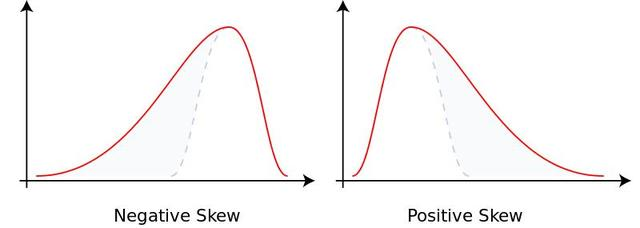• ## 集中趋势

千次阅读 2016-07-02 16:20:21
统计学分类统计学可以分成两类： 描述性统计学 有一堆数据, 希望在不告诉别人所有数据的情况下介绍这些数据的情况，可以通过找到...数据集中趋势集中趋势（central tendency）: 一组数字的集中趋势，也叫“平均数（a
统计学分类
统计学可以分成两类：
描述性统计学

有一堆数据, 希望在不告诉别人所有数据的情况下介绍这些数据的情况，可以通过找到一些指示性的数字来代表所有的数据。
推断统计学

运用数据来对事物做结论，例如从总体中得到一个样本，对样本做一些数学运算，就可以推断出总体的整体情况。

我们从描述性统计学开始学习。
数据集中趋势
集中趋势（central tendency）: 一组数字的集中趋势，也叫“平均数（average）”。这里的平均数和我们常见的均值不一样，是广义上的平均数，表示能反应一组数据集中趋势的数字。包括如下统计量：
均值（mean）

定义：均值有很多种，我们这里指的仅仅是算数平均数（arithmetic mean）。我们还知道有调和平均数（harmonic mean）  计算公式：

Meann=a1+a2+a3+...+ann

Mean_n = \frac{a_1 + a_2 + a_3 + ... + a_n}{n}
其中

an
$a_n$是样本的元素，

n
$n$是样本个数。

中位数（median）

定义：对于有限的数集，可以通过把所有观察值高低排序后找出正中间的一个作为中位数。如果观察值有偶数个，通常取最中间的两个数值的平均数作为中位数。
计算公式：
X1$X_1$,…,

XN
$X_N$从小到大排列顺序为：

X(1)
$X_{(1)}$,…,

X(N)
$X_{(N)}$

N
$N$为奇数的时候：
MedianN=X(N+12)Median_N = X_{(\frac{N + 1}{2})}

N
$N$为偶数的时候：
MedianN=XN2+XN2+12Median_N = \frac{ X_{\frac{N}{2}} + X_{\frac{N}{2} + 1}}{2}
众数（mode）

定义：在统计分布上具有明显集中趋势点的数值，代表数据的一般水平（众数可以不存在或多于一个）。  修正定义：是一组数据中出现次数最多的数值，叫众数，有时众数在一组数中有好几个。  理性理解：简单的说，就是一组数据中占比例最多的那个数。
极差（range）

定义：最大值与最小值之差。它是标志值变动的最大范围。
方差 (variance)

定义：方差是实际值与期望值之差平方的平均值，方差是在概率论和统计方差衡量随机变量或一组数据是离散程度的度量.  计算公式：
样本减去期望的平方的期望

s2=1n[(x1−x)2+(x2−x)2+...+(xn−x)2]

s^2 = \frac{1}{n}[{(x_1 - x)}^2 + {(x_2 - x)}^2 + ... + {(x_n - x)}^2]平方的期望减去期望的平方

D(x)=E(x2)−(E(x))2

D(x) = E(x^2) -{(E(x))}^2
中程数 (midrange)

定义：最大值与最小值的算数平均数  计算公式：

Midrange=Xmax+Xmin2

Midrange = \frac{X_{max} + X_{min}}{2}

动手写代码
样例数据
数据样本a: 1 1 2 3 4数据样本b: 1 1 2 3 4 4
Python 实现
# -*- coding: utf-8 -*-
"""
Created on Sat Jul 02 15:31:43 2016

@author: zang
"""
import time;
import random;
class Math:
#求极差
@staticmethod
def range(l):
return max(l)-min(l)
#求中程数
@staticmethod
def midrange(l):
return float(max(l) + min(l))/2
#求均值
@staticmethod
def mean(l):
return float(sum(l))/len(l)
#求中位数
@staticmethod
def median(l):
l=sorted(l);#先排序
if len(l)%2 == 1:
return l[len(l)/2]
else:
return (l[len(l)/2-1]+l[len(l)/2])/2.0
#求众数
@staticmethod
def mode(l):
#统计list中各个数值出现的次数
count_dict = {}
for i in l:
count_dict[i] = count_dict.get(i,0) + 1
#求出现次数的最大值
max_appear = 0
for v in count_dict.values():
if v > max_appear:
max_appear = v
if max_appear == 1:
return "no mode in input data!"
mode_list = []
for k,v in count_dict.items():
if v == max_appear:
mode_list.append(k)
return mode_list
#求方差
@staticmethod
def variance(l):#平方的期望-期望的平方
s1 = 0;
s2 = 0;
for i in l:
s1 += i**2
s2 += i
return float(s1)/len(l)-(float(s2)/len(l))**2

#求方差2
@staticmethod
def variance2(l):#样本-期望的平方的期望
ex = float(sum(l))/len(l);
s=0;
for i in l:
s += (i-ex)**2;
return float(s)/len(l)
sa = [1,1,2,3,4]
sb = [1,1,2,3,4,4]
print "sa极差为：{0}".format(Math.range(sa))
print "sa中程数为：{0}".format(Math.midrange(sa))
print "sa均值为：{0:.2f}".format(Math.mean(sa))
print "sa中位数为：{0}".format(Math.median(sa))
print "sa众数为：{0}".format(Math.mode(sa))
print "sa方差为：{0:.2f}".format(Math.variance(sa))
print "sa方差为：{0:.2f}".format(Math.variance2(sa))
sa极差为：3
sa中程数为：2.5
sa均值为：2.20
sa中位数为：2
sa众数为：
sa方差为：1.36
sa方差为：1.36

print "sb极差为：{0}".format(Math.range(sb))
print "sb中程数为：{0}".format(Math.midrange(sa))
print "sb均值为：{0:.2f}".format(Math.mean(sb))
print "sb中位数为：{0}".format(Math.median(sb))
print "sb众数为：{0}".format(Math.mode(sb))
print "sb方差为：{0:.2f}".format(Math.variance(sb))
print "sb方差为：{0:.2f}".format(Math.variance2(sb))
sb极差为：3
sb中程数为：2.5
sb均值为：2.50
sb中位数为：2.5
sb众数为：[1, 4]
sb方差为：1.58
sb方差为：1.58

两种方差计算方法性能比较
arraylist=[];
for i in range(1,1000000):
arraylist.append(i);
random.shuffle(arraylist);
time_start=time.time();
print "方差为：{0:.2f}".format(Math.variance(arraylist));
time_end=time.time();
print "{0}s".format(time_end-time_start);
time_start=time.time();
print "方差为：{0:.2f}".format(Math.variance2(arraylist));
time_end=time.time();
print "{0}s".format(time_end-time_start);  
方差为：83333166666.67
1.35199999809s
方差为：83333166666.21
0.457999944687s

统计量对数据集中趋势的描述
到底哪个统计量能很好的描述数据呢，其实实际中是多个统计量一起考虑，才能从多个角度去反映数据的特性。举个例子。
数据样本sc: 3 3 3 3 3 100
其中有个离群值：100
sc= [3,3,3,3,3,100]
print "sc极差为：{0}".format(Math.range(sc))
print "sc中程数为：{0}".format(Math.midrange(sc))
print "sc均值为：{0:.2f}".format(Math.mean(sc))
print "sc中位数为：{0}".format(Math.median(sc))
print "sc众数为：{0}".format(Math.mode(sc))
print "sc方差为：{0:.2f}".format(Math.variance(sc))
print "sc方差为：{0:.2f}".format(Math.variance2(sc))
sc极差为：97
sc中程数为：51.5
sc均值为：19.17
sc中位数为：3.0
sc众数为：
sc方差为：1306.81
sc方差为：1306.81

从结果可以看出，极差，中程数，均值都收到离群值的影响，有所偏移。  离群值目前没有直接的定义，通常离群值是异于数据样本其他值的数值，离群值的产生来源于测量误差和其他原因。
参考资料
yongh701 CSDN博客可汗学院公开课 网易公开课
展开全文统计学 Python 数学
• 八年级数学下册第20章数据的初步分析20.2数据的集中趋势与离散程度20.2.1数据的集中趋势作业设计新版沪科版
• 此时我们主要用到两个统计学工具：集中趋势和离散趋势。 1. 集中趋势 集中趋势是一组数据的代表值，那用什么值作代表最有代表性呢？当然这个值应该和所有值差距不大是最好，此时我们首先想到的就是平均数，事实上，...
给定一组数据，我们怎么来判断业务的基本情况呢？此时我们主要用到两个统计学工具：集中趋势和离散趋势。
1. 集中趋势
集中趋势是一组数据的代表值，那用什么值作代表最有代表性呢？当然这个值应该和所有值差距不大是最好，此时我们首先想到的就是平均数，事实上，用来衡量集中趋势的最常用指标就是平均数，当然有时我们也使用中位数。
平均数和中位数一般是不同的，除非样本呈正态分布。如果衡量集中趋势的指标选择不合理，那么对业务整体情况的判断往往会出现争议，最常见的例子就是“工资水平”统计数据的梗——大多数人总是感到“拖了大家的后腿”。为什么会这样呢？因为工资收入是偏态分布的，而且是正偏态分布——大多数人工资处于较低的水平。
那么表示集中趋势时，什么时候选择平均数，什么时候选择中位数呢？我们可以通过考察数据分布的正态、偏态情况进行选择。如果样本呈正态分布，那么集中趋势使用平均数或中位数表示均可，因为两者是相等的。 如果样本呈偏态分布，那么选择中位数更能反映数据的集中趋势。通常情况下，正偏态的中位数小于平均数，负偏态的中位数大于平均数。因此，如果工资水平的计量采用中位数，大家心里接受的程度可能会更高一些。 比如以下工资水平的抽样数据，用中位数表示是3000，用平均数表示是9200。显然选择中位数的表示集中趋势更符合实际。 salary=[2500,3500,2000,4000,2200,3000,1800,20000,50000,3000]
2. 离散趋势
离散趋势反映了样本数据之间的差异水平。反映离散趋势的统计指标一般包括标准差/方差、极差、四分位间距IQR和变异系数。
极差是样本最大值与最小值的差；四分位间距IQR是75%分位数与25%分位数的差，显然四分位间距IQR一般要比极差小；变异系数是标准差与均值的比值，通常认为如果变异系数超过15%，则说明业务状况是很不稳定的。上例中，工资样本的变异系数是1.58，说明工资水平是极不稳定的。
除了变异系数是相对量化指标外，其它三个指标都是绝对量化指标。因此，变异系数可以进行不同数据集离散程度的比较，而其它三个指标不可以，因为不同数据集的数据尺度有所差异。
集中趋势和离散趋势相结合才能更准确的反映业务状况，当离散趋势不明显时，集中趋势反映总体水平的能力就越强。
推荐自编课程《零基础学python数据分析》 推荐自编简明预测分析教程(Python版)
展开全文数据分析 机器学习 统计学 离散趋势
• 集中趋势 (central tendency) 一组数据向其中心值靠拢的倾向和程度 测度集中趋势就是寻找数据水平的代表值或中心值 不同类型的数据用不同的集中趋势测度值 低层次数据的测度值适用于高层次的测量数据，但高层次数据...
集中趋势 (central tendency)
一组数据向其中心值靠拢的倾向和程度测度集中趋势就是寻找数据水平的代表值或中心值不同类型的数据用不同的集中趋势测度值低层次数据的测度值适用于高层次的测量数据，但高层次数据的测度值并不适用于低层次的测量数据

集中趋势的度量
分类数据：众数（mode）1. 众数2. 众数 (不惟一性)

顺序数据：中位数和分位数1. 中位数 (median)2. 四分位数 (quartile)

数值型数据：平均数1. 简单平均数 (Simple mean)2. 加权平均数 (Weighted mean)3. 几何平均数 (geometric mean)

众数、中位数和平均数的比较1. 众数、中位数和平均数的关系2. 众数、中位数、平均数的特点和应用

分类数据：众数（mode）
1. 众数
一组数据中出现次数最多的变量值适合于数据量较多时使用不受极端值的影响一组数据可能没有众数或有几个众数主要用于分类数据，也可用于顺序数据和数值型数据
2. 众数 (不惟一性)
无众数 原始数据: 10 5 9 12 6 8一个众数 原始数据: 6 5 9 8 5 5多于一个众数 原始数据: 25 28 28 36 42 42
顺序数据：中位数和分位数
1. 中位数 (median)
排序后处于中间位置上的值不受极端值的影响主要用于顺序数据，也可用数值型数据，但不能用于分类数据各变量值与中位数的离差绝对值之和最小
2. 四分位数 (quartile)
排序后处于25%和75%位置上的值不受极端值的影响
数值型数据：平均数
平均数(mean)
也称为均值集中趋势的最常用测度值一组数据的均衡点所在体现了数据的必然性特征易受极端值的影响有简单平均数和加权平均数之分根据总体数据计算的，称为平均数；根据样本数据计算的，称为样本平均数。
1. 简单平均数 (Simple mean)
2. 加权平均数 (Weighted mean)
3. 几何平均数 (geometric mean)
n 个变量值乘积的 n 次方根适用于对比率数据的平均主要用于计算平均增长率
众数、中位数和平均数的比较
1. 众数、中位数和平均数的关系
2. 众数、中位数、平均数的特点和应用
（1）众数
不受极端值影响具有不惟一性数据分布偏斜程度较大且有明显峰值时应用
（2）中位数
不受极端值影响数据分布偏斜程度较大时应用
（3）平均数
易受极端值影响数学性质优良数据对称分布或接近对称分布时应用
展开全文大数据 数据分析 统计学
• 集中趋势分析主要靠平均数、中数、众数等统计指标来表示数据的集中趋势。例如测试班级的平均成绩是多少？是正偏分布还是负偏分布？ 离中趋势分析主要靠全距、四分差、平均差、方差、标准差等统计指标来研究数据的...
集中趋势分析主要靠平均数、中数、众数等统计指标来表示数据的集中趋势。例如测试班级的平均成绩是多少？是正偏分布还是负偏分布？
离中趋势分析主要靠全距、四分差、平均差、方差、标准差等统计指标来研究数据的离中趋势。例如，当我们想知道两个教学班的语文成绩，哪个班级的成绩分布更分散时，就可以用两个班级的四分差或百分点来比较。

注：四分差
1.四分位差（quartile deviation），它是上四分位数（QU，即位于75%）与下四分位数（QL，即位于25%）的差的平均值。 2.计算公式为：Qd  = （QU-QL） / 2 3.四分位差反映了中间50%数据的离散程度，其数值越小，说明中间的数据越集中；其数值越大，说明中间的数据越分散。四分位差不受极值的影响。此外，由于中位数处于数据的中间位置，因此，四分位差的大小在一定程度上也说明了中位数对一组数据的代表程度。四分位差主要用于测度顺序数据的离散程度。对于数值型数据也可以计算四分位差，但不适合分类数据。 4.四分位数是将一组数据由小到大（或由大到小）排序后，用3个点将全部数据分为4等份，与这3个点位置上相对应的数值称为四分位数，分别记为Q1（第一四分位数）、Q2（第二四分位数，即中位数）、Q3（第三四分位数）。其中，Q3到Q1之间的距离的差的一半又称为四分位差，记为Q。四分位差越小，说明中间部分的数据越集中；四分位差越大，则意味着中间部分的数据越分散。 
展开全文• 集中趋势体现了数据的一般水平，可以用来快速了解样本数据的概况。 二、分类 集中趋势指标包括平均值、中位数、众数 平均值：是最常用的集中趋势指标，但有弊端，单纯的计算平均值具有一定的误导性，对异常数据不...数据分析
• 文华财经指标公式源码期货软件指标赢顺云指标集中趋势指标.doc文档
• 文华财经指标公式源码WH6指标公式赢顺云集中趋势指标.doc文档
• 文华财经期货软件指标公式源码期货指标赢顺云集中趋势指标.doc文档
• 数据描述的第一个维度是数据的集中趋势描述。数据的集中趋势描述是寻找反应事物特征的数据集合的代表值或中心值，这个代表值或中心值可以很好反映事物目前所处的位置和发展水平，通过对事物集中趋势指标的多次测量和...数据分析
• 文华财经指标公式源码WH6指标公式期货软件指标集中趋势指标.doc文档
• 文华财经期货软件指标公式源码期货指标赢顺云指标集中趋势指标.doc文档
• 2021年高中数学新人教A版必修第二册　9.2.2总体集中趋势的估计　课件
• ###什么是集中趋势度量？ A measure of central tendency (also referred to as measures of centre or central location) is a summary measure that attempts to describe a whole set of data with a single ...
• 商贸零售行业周报：2020Q1零售板块基金配置提升，龙头集中趋势明显.pdf
•数学建模
• 2015春八年级数学下册《20.2.1 数据集中趋势》课件4 （新版）沪科版
• 2015春八年级数学下册《20.2.1 数据集中趋势》课件2 （新版）沪科版
• 2015春八年级数学下册《20.2.1 数据集中趋势》课件3 （新版）沪科版
• 2015春八年级数学下册《20.2.1 数据集中趋势》课件1 （新版）沪科版
• 自由度，英文称degree of freedom，简称DF，是指...集中趋势，英文称central tendency，是指一组数据向某一中心值靠拢的程度，它反映了一组数据中心点的位置所在。其在中心附近的观察值数目较多，远离中心的较少，常统计学基本概念
• 2020版经营者集中趋势与发展之中国篇（英文版）精品报告2020.pdf
• 建筑材料行业周报：消费建材龙头集中趋势未变，建议关注优质成长细分龙头.pdf
• 算法以机器筛选得到的主成分作为弱分类器集，通过集中趋势的方法改进了自适应增强技术。这样既避免了过拟合问题，也解决了弱分类器叠加错误陷阱。实验表明该算法能有效地应用于英语作文智能评分系统，且与人工评分......